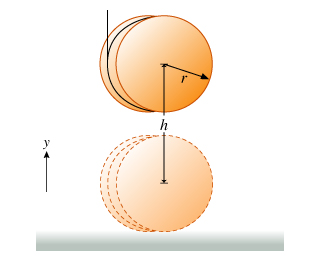# PERIOD OF THE LEG The period of the leg can be approximated by treating the leg as a physical pendulum, with a period of 2pi*sqrt(I/mgh), where I is the moment of inertia, m is the mass, and h is the distance from the pivot point to the center of mass

PERIOD OF THE LEG
The period of the leg can be approximated by treating the leg as a physical pendulum, with a period of 2pi*sqrt(I/mgh), where I is the moment of inertia, m is the mass, and h is the distance from the pivot point to the center of mass.
The leg can be considered to be a right cylinder of constant density. For a man, the leg constitutes 16% of his total mass and 48% of his total height.
Find the period of the leg of a man who is 1.82 m in height with a mass of 72 kg. The moment of inertia of a cylinder rotating about a perpendicular axis at one end is ml^2/3

This Homework Help Question: "PERIOD OF THE LEG The period of the leg can be approximated by treating the leg as a physical pendulum, with a period of 2pi*sqrt(I/mgh), where I is the moment of inertia, m is the mass, and h is the distance from the pivot point to the center of mass" No answers yet.

We need 10 more requests to produce the answer to this homework help question. Share with your friends to get the answer faster!

0 /10 have requested the answer to this homework help question.

Once 10 people have made a request, the answer to this question will be available in 1-2 days.
All students who have requested the answer will be notified once they are available.

#### Earn Coin

Coins can be redeemed for fabulous gifts.

Similar Homework Help Questions
• ### A uniform circular disk whose radius R is 32.0 cm is suspended as a physical pendulum from a point on its rim

A uniform circular disk whose radius R is 32.0 cm is suspended as a physical pendulum from a point on its rim. (a) What is its period of oscillation? __ s (b) At what radial distance r < R is there a point of suspension that gives the same period? __ cm in the book.. it gives me a hint: ..... the period of oscillation is given by T = 2pi sqrt(I/mgh), where I is the rotational inertia of the...

• ### A cylinder with moment of inertia I about its center of mass, mass m, and radius r has a string wrapped around it whi...A cylinder with moment of inertia I about its center of mass, mass m, and radius r has a string wrapped around it which is tied to the ceiling (Figure 1) . The cylinder's vertical position as a function of time is y(t). At time t=0 the cylinder is released from rest at a height h above the ground. Part B In similar problems involving rotating bodies, you will often also need the relationship between angular acceleration, ?, and linear...

• ### A physical pendulum of 1 kg of mass oscillates in a simple harmonic movement, with a period of π sec. The distance from the center of mass to the axis of rotation is 40 cm. What is your moment of iner...

A physical pendulum of 1 kg of mass oscillates in a simple harmonic movement, with a period of π sec. The distance from the center of mass to the axis of rotation is 40 cm. What is your moment of inertia with respect to the center of mass? (consider g = 10 m/s^2)? a) 0.66 kg•m2 b) 1 kg•m2 c) 0.46 kg•m2             d) 0.84 kg•m2              e) 1.16 kg•m2

• ### 1) The parallel axis theorem provides a useful way to calculate the moment of inertia I...

1) The parallel axis theorem provides a useful way to calculate the moment of inertia I about an arbitrary axis. The theorem states that I = Icm + Mh2, where Icm is the moment of inertia of the object relative to an axis that passes through the center of mass and is parallel to the axis of interest, M is the total mass of the object, and h is the perpendicular distance between the two axes. Use this theorem and...